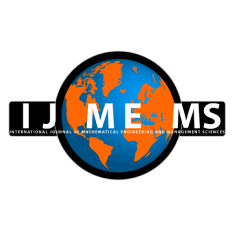### International Journal of Mathematical, Engineering and Management Sciences

#### ISSN: 2455-7749 . Open Access

A Solution Approach for Multi-Level Multi-Objective Quadratic Fractional Programming with Trapezoidal Fuzzy Numbers using Rouben Ranking Function

#### A Solution Approach for Multi-Level Multi-Objective Quadratic Fractional Programming with Trapezoidal Fuzzy Numbers using Rouben Ranking Function

Vandana Goyal
Department of Mathematics, Maharishi Markandeshwar (Deemed to be University), Mullana, Ambala, India.

Namrata Rani
Department of Mathematics, Maharishi Markandeshwar (Deemed to be University), Mullana, Ambala, India.

Deepak Gupta
Department of Mathematics, Maharishi Markandeshwar (Deemed to be University), Mullana, Ambala, India.

;
Accepted on January 04, 2022

Abstract

The paper proposed a methodology for obtaining a set of efficient solutions for a model which is multi-level multiobjective quadratic with fractional objectives and constraints having trapezoidal fuzzy number (MLMOQFP-TrFN) as coefficients. The model consists of r-levels with several objectives involved to be solved under a set of quadratic constraints. The proposed approach starts with the solution process of the top level and other levels are solved in succession but depending on the solution of the previous levels. The solution process of each level comprises mainly three stages. In the beginning, the Rouben Ranking Function is used to convert the rth-level of fuzzy model into a deterministic or crisp one. After that, the crisp form is reconstructed to get a non-fractional model with the help of an iterative parametric approach. Further, in the last, non-fractional model which is still having multiple objectivesis reconstructed to form a model having only one objective with ɛ -constraint method and is lastly solved by following the solution of (r-1)th- level to get a desired set of efficient solution. Such programming models are very useful in day to day life such as in economic planning, industrial activities, waste management, neural networking, unmanned aerial and underwater vehicle management, agricultural yield improvement, transportation problems with maximizing profits and minimizing wastage of material and cost and so on. An algorithm depicting all the steps of solution approach is also presented to reflect a clear idea for the approach. In addition, a numerical regarding the presentation of complete approach that is studied is given in the end.

Keywords- ɛ-constraint method, Parametric approach, Rouben ranking function, Multi-level multiobjective quadratic fractional programming model (MLMOQFP), Trapezoidal fuzzy number (TrFN)

Citation

Goyal, V., Rani, N., & Gupta, D. (2022). A Solution Approach for Multi-Level Multi-Objective Quadratic Fractional Programming with Trapezoidal Fuzzy Numbers using Rouben Ranking Function. International Journal of Mathematical, Engineering and Management Sciences, 7(1), 131-152. https://doi.org/10.33889/IJMEMS.2022.7.1.009.